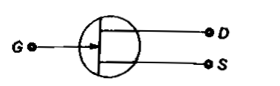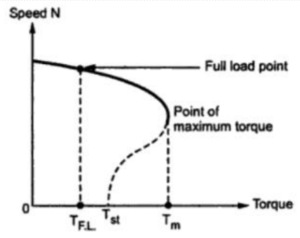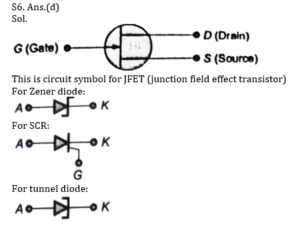Engineering Jobs   »   UPRVUNL-JE 2021, UPRVUNL-JE ELECTRICAL, uprvunl je...

# UPRVUNL’21 EE: Daily Practices Quiz 23-Aug-2021

UPRVUNL JE Recruitment 2021:

A total of 196 vacancies were released by the UPRVUNL Recruitment 2021. The selection process will be based on the written examination which will be divided into two parts – Part I and Part II. Part I will have 150 objective-type questions from the syllabus for the Diploma Engineering in the relevant branch. While Part II will have 50 objective-type questions comprising General Knowledge, General Hindi, and Reasoning.

UPRVUNL-JE ELECTRICAL-2021 QUIZ

Each question carries 1 mark.

Negative marking: 1/4 mark
Total Questions: 06
Time: 06 min.

Q1. In comparison to steam plant, the annual fixed cost of nuclear plant is
(a) Quite high
(b) Quite low
(c) almost Equal
(d) Can’t Say

Q2. The area under the load duration curve represents…
(a) the average load of the system
(c) maximum demand of the power station
(d) energy generated in the period considered

Q3. Speed of induction motor at rated torque is ____ than at maximum torque.
(a) greater
(b) same
(d) lesser

Q4. Calculate torque developed by the 3-phase, 50Hz, 5 H.P., 4 pole induction motor runs at 1500 rpm
(a) 23.5 N-m
(b) 22 N-m
(c) 0 N-m
(d) 10 N-m

Q5. A CRO (Cathode Ray Oscilloscope) can be used to measure-
(a) Ac voltage
(b) Dc voltage
(c) Frequency
(d) All of the above

Q6. Figure represents a –(a) Zener diode
(b) Silicon controlled rectifier
(c) Tunnel diode
(d) Junction field effect transistor

SOLUTIONS
S1. Ans.(a)
Sol. In comparison to steam plant, the annual fixed cost of nuclear plant is Quite high.
The fixed costs of power generation are essentially capital costs and land.

S2. Ans.(d)
Sol. The load duration curve is the plot between load and time. The load duration curve is constructed using the daily load curve. The area under the load duration curve represents the total energy generated by the utility’s generating system in the considered period.

S3. Ans.(a)
Sol. Speed of induction motor at rated torque is greater than at maximum torque.S4. Ans.(c)
Sol. ACQ; N_r=1500 rpm & N_s=120f/P=(120×50)/4=1500 rpm
If the rotor rotates at the synchronous speed, then the torque developed by the motor is zero.

S5. Ans.(d)
Sol. A Cathode Ray Oscilloscope is used for the measurement of AC as well as DC current. It is used for the calculation peak to peak voltage, r.m.s value, duty cycle etc. The radio frequency, audio frequency and intermediate frequency signals can be measured by making use of a CRO.Sharing is caring!

Thank You, Your details have been submitted we will get back to you.